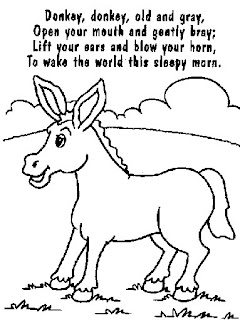## Thursday, 7 August 2008

### Out of the Mouth of a Donkey?Got an e-mail a couple of days ago from an Indian Mathematician named Sajeev Singh. Sanjeev asked me to let people know about his work at Total Gadha where he posts notes about mathematical ideas with some comments etc. I took a look and found some really well done posts on some interesting ideas about math that is accessible to high-school and college age folks. Gadha, by the way, if my memory serves me in old age, means Donkey, but I'm not sure how that reflects in the local culture. One more reason to visit India some day.

One of the notes he posted took me back, yet again, to the question about the 6! or 720 six-digit numbers that can be made using only the digits 1,2,3,4,5, and 6 once each. I realized that with a single brief mental calculation you could confirm that a) none of them were prime, and b) none of them were perfect squares. Why NOT?? (think, then read)

The reason has to do with the digit root; what used to be called casting-out-nines(and some of us still do call it that). If you add the digits, 1+2+3+4+5+6 the total is 21, and if you add 2+1 you get 3, so the remainder when any of the 720 numbers is divided by nine is going to be three, try it. 243561, for example, when divided by nine has a quoatient of 27062 with a remainder of three. Any number that has a digit root of three is divisible by three. All 720 of the six-digit arrangements are divisible by three. But how do I know none of them is a perfect square???? Same answer, the digit root is 3. THe digit root of a square number is the square of the digit root of the square root. Let's look at some numbers and their squares:

12 = has digit root of one

22=has digit root of four

32=digit root of nine

42=16, so digit root of seven

52=25 so digit root of seven

62=36 so digit root of nine

72=49 so digit root of four

82=64 so digit root of one

92=81 so digit root is nine

Keep goin.. these are the only four numbers you get for the digit root of a square,... ever...square 37?? digit root is one... square 155?... digit root is four.. you do these in your head.. To find the digit root of the square of 23 you add the 2 and 3 to get five, so the digit root of 23 is five. That means the digit root of 23 squared is the digit root of 5 squared.... seven. 23 2 = 529. Add the digits you get 16 and add those digits you get...yep, 7. What does NOT ever happen is a digit root of 3; so none of the 720 numbers are square numbers. Can you prove than none of them are perfect CUBES????

Two things better than being reminded of something you once knew are to learn something you never knew, and even better, to be stimulated by a question to discover something new on your own.... and both those things happened after going to TotalGahda. Rather than throw all that at you and once though, I will give you a chance to experiment with one.

Think of a two digit number, now write it down three times xyxyxy and divide by 7. What is the remainder? Wait, divide it by 13.... NOw what is the remainder? I just discovered this morning that this works.. and proved a general version of it which I will include next time with a conjecture about a couple of extensions to all primes... not sure if or who might have done it before... and I can add that if you write the two digit number out eight times, it will be divisible by 17. It will also work if you write down any four digit number four times, or any eight digit number twice (wow, what a clue)

If you have trouble dividing big long numbers and finding the remainder, try using the Google search engine. Just enter something like "4512451245124512 mod 17 =" in the search box and the Google calculator takes over and gives you the remainder (modulus)

Ok, IF you get stuck on that one, you can try this, write down any THREE digit number, and copy it again...xyzxyz to make a number... now divide by seven again, and by 13???? The reason this one works may be a little easier(I've know it has been around in calculator books before).... see if you can figure it out. I'll be back in a couple of days with the additional material, and if you KNOW this has been done already, especially if you have a citation, Please advise.